# Texas Go Math Grade 1 Lesson 2.4 Answer Key Greater Than or Less Than

Refer to our Texas Go Math Grade 1 Answer Key Pdf to score good marks in the exams. Test yourself by practicing the problems from Texas Go Math Grade 1 Lesson 2.4 Answer Key Greater Than or Less Than.

## Texas Go Math Grade 1 Lesson 2.4 Answer Key Greater Than or Less Than

Essential Question
How can you decide if a number is greater than or less than another number?
Explanation:
Compare the tens if tens are equal we have to compare the ones.

Explore
UseDraw quick pictures to show your work.For The Teacher

• Have children draw tens and ones to show each number. Then have them generate a number that makes the sentence true. Have them draw the number they chose.
Explanation:Math Talk
Mathematical Processes
How can tens and ones help you decide which number is greater and which number is less?
Explanation:
Compare the tens if tens are equal we have to compare the ones.

Model and Draw

Use place value to compare a number to another number. Write a number to compare.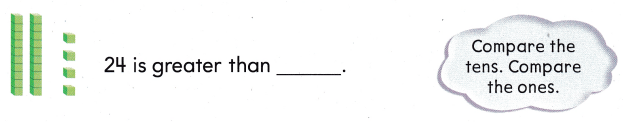Explanation: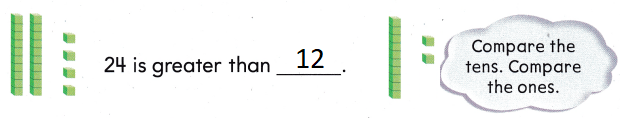24 is greater than 12
2 is greater than 1
4 is greater than 2

Compare the tens. Compare the ones.

___ is greater than 52.64 is greater than 52
6 is greater than 5 and 4 is greater than 2

Share and Show

Use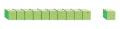and place value to show each number.

Question 1.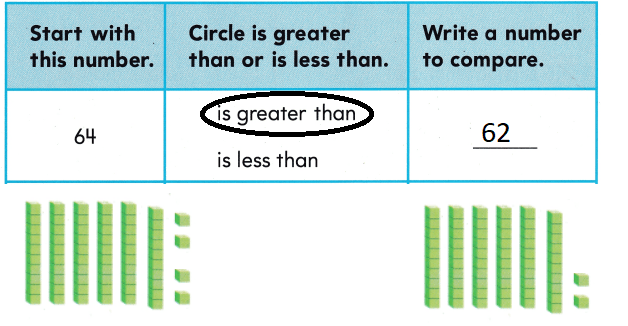Explanation:
64 is greater than 62
64 > 62

Question 2.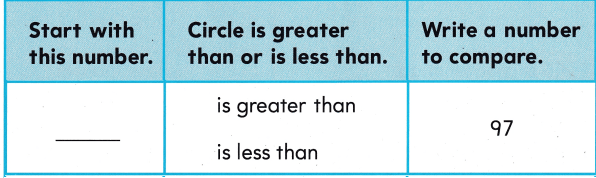Explanation:
68 is less than 97
68 < 97

Problem Solving

Circle is greater than or is less than. Write a number to compare.

Question 3.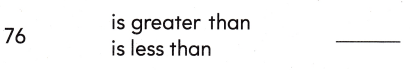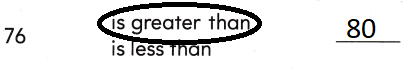Explanation:
80 is greater than 76
80 > 76

Question 4.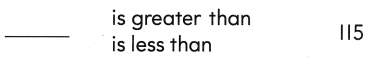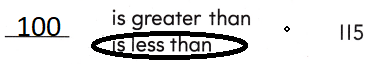Explanation:
100 is lesser than 115
100 < 115

Solve. Write the number.

Question 5.
I am a number greater than 54. I have fewer tens than 90.
_____
65
Explanation:
The number might be in 55 and 89

Question 6.
H.O.T. I am a number less than 36. I am greater than 24.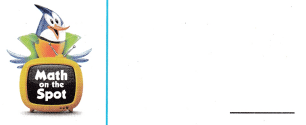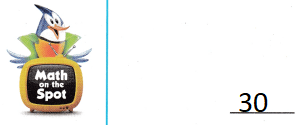Explanation:
I am a number less than 36.
I am greater than 24.
the number be 30

Question 7.
H.O.T. Multi-Step I am a number less than 8 tens 5 ones. I am greater than 77. I have fewer ones than 5 ones. I am not 83.
_____
82
Explanation:
82 is less than 85
and not 83
that is 82

Question 8.
Multi-Step The rabbits have 56 wheels. The monkeys have more wheels. Which could be the number of wheels the monkeys have?
(A) 50
(B) 65
(C) 55
Explanation:
65 be the number of wheels the monkeys have

Question 9.
Communicate There are 4-8 cars on the street. Which number is greater than the number of cars?(A) 52
(B) 39
(C) 44
Explanation:
52 is greater than the number of cars 48

Question 10.
Alex writes the number 87. Which number is less than Alex’s number?
(A) 77
(B) 88
(C) 96
Explanation:
77 is less than Alex’s number 87
77 < 87

Question 11.
Texas Test Prep Which of these is less than 4 tens?
(A) 50
(B) 22
(C) 41
Explanation:
22 is less than 4 tens
2 < 4

Take Home Activity

• Have your child choose a number that is greater than 88. Then have him or her choose a number that is less than 93.
88 is the number
The number greater  than 88 is 90
And the number less than 93 is 92

### Texas Go Math Grade 1 Lesson 2.4 Homework and Practice Answer Key

Use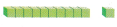and place value to show each number.

Question 1.Explanation:
10 is lesser than 12
10 < 12

Question 2.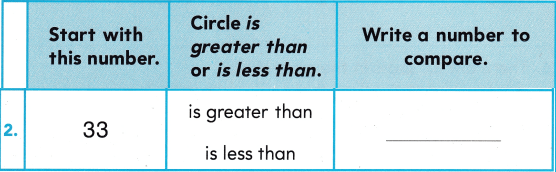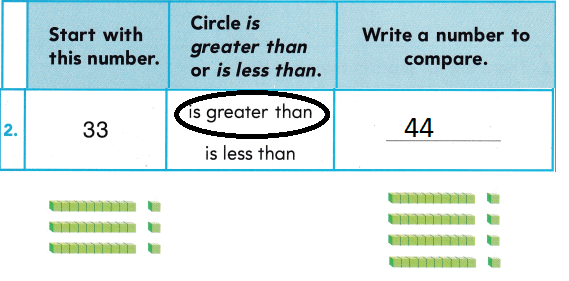Explanation:
33 is lesser than 44
3 < 4

Circle is greater than or is less than.
Write a number to compare.

Question 3.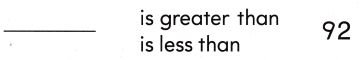Explanation:
86 is less than 92.
8 < 9

Solve. Write the number.

Question 4.
Multi-Step Aya picks a number greater than 3 tens 6 ones. The number is less than 52. It has more than 6 ones. What could the number be?
6 + 6 = 12
36 + 6 = 42
Explanation:
42 is the number.

Texas Test Prep

Lesson Check

Question 5.
Miss Kent has 23 eggs. Mrs. Jones has more eggs. Which number of eggs could Mrs. Jones have?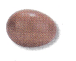(A) 36
(B) 12
(C) 20
Explanation:
36 is the  number of eggs that Mrs. Jones have

Question 6.
There are 82 seats in the gym. Which number is greater than the number of seats?
(A) 76
(B) 80
(C) 91
Explanation:
91 number is greater than the number of seats
9 > 8

Question 7.
Ed writes the number 44? Which number is less than Ed’s number?
(A) 55
(B) 33
(C) 77
Explanation:
33 is the number is less than Ed’s number

Question 8.
Which number is greater than 6 tens?
(A) 72
(B) 59
(C) 30
Explanation:
7 > 6
72 is greater than 6 tens

Question 9.
Which number is less than 6 tens?
(A) 62
(B) 59
(C) 76